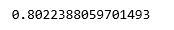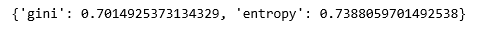GeeksforGeeks App
Open AppBrowser
Continue

# ML | Logistic Regression v/s Decision Tree Classification

Logistic Regression and Decision Tree classification are two of the most popular and basic classification algorithms being used today. None of the algorithms is better than the other and one’s superior performance is often credited to the nature of the data being worked upon.

We can compare the two algorithms on different categories –

As a simple experiment, we run the two models on the same dataset and compare their performances.

Step 1: Importing the required libraries

## Python3

 `import` `numpy as np``import` `pandas as pd``from` `sklearn.model_selection ``import` `train_test_split``from` `sklearn.linear_model ``import` `LogisticRegression``from` `sklearn.tree ``import` `DecisionTreeClassifier`

Step 2: Reading and cleaning the Dataset

## Python3

 `cd C:\Users\Dev\Desktop\Kaggle\Sinking Titanic``# Changing the working location to the location of the file``df ``=` `pd.read_csv(``'_train.csv'``)``y ``=` `df[``'Survived'``]` `X ``=` `df.drop(``'Survived'``, axis ``=` `1``)``X ``=` `X.drop([``'Name'``, ``'Ticket'``, ``'Cabin'``, ``'Embarked'``], axis ``=` `1``)` `X ``=` `X.replace([``'male'``, ``'female'``], [``2``, ``3``])``# Hot-encoding the categorical variables` `X.fillna(method ``=``'ffill'``, inplace ``=` `True``)``# Handling the missing values`

Step 3: Training and evaluating the Logistic Regression model

## Python3

 `X_train, X_test, y_train, y_test ``=` `train_test_split(``            ``X, y, test_size ``=` `0.3``, random_state ``=` `0``)` `lr ``=` `LogisticRegression()``lr.fit(X_train, y_train)``print``(lr.score(X_test, y_test))`Step 4: Training and evaluating the Decision Tree Classifier model

## Python3

 `criteria ``=` `[``'gini'``, ``'entropy'``]``scores ``=` `{}` `for` `c ``in` `criteria:``    ``dt ``=` `DecisionTreeClassifier(criterion ``=` `c)``    ``dt.fit(X_train, y_train)``    ``test_score ``=` `dt.score(X_test, y_test)``    ``scores ``=` `test_score` `print``(scores)`On comparing the scores, we can see that the logistic regression model performed better on the current dataset but this might not be the case always.

My Personal Notes arrow_drop_up